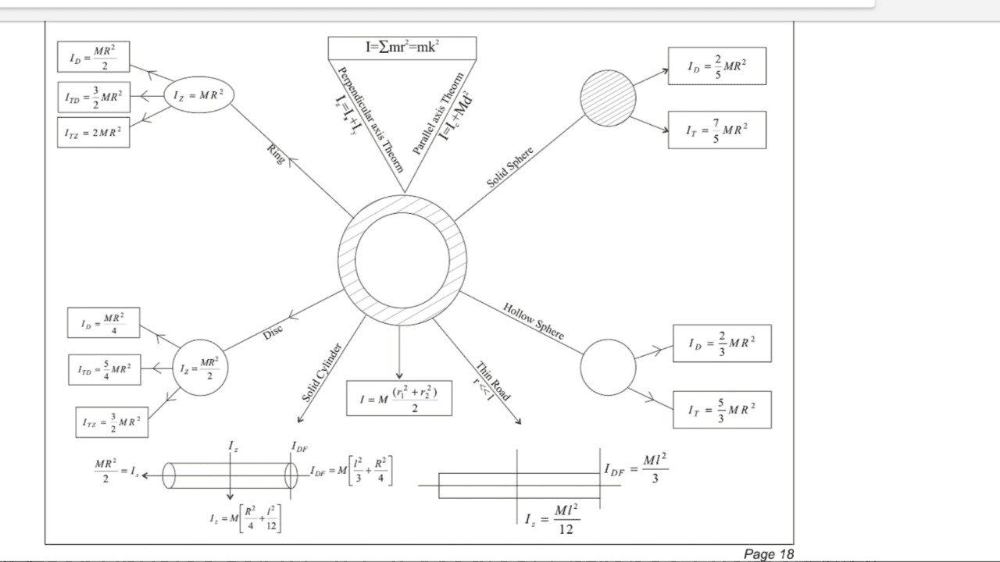# Moment of inertia Notes | Study Physics For JEE - JEE

## JEE: Moment of inertia Notes | Study Physics For JEE - JEE

The document Moment of inertia Notes | Study Physics For JEE - JEE is a part of the JEE Course Physics For JEE.
All you need of JEE at this link: JEEThe moment of inertia, otherwise known as the angular mass or rotational inertia, of a rigid body is a quantity that determines the torque needed for a desired angular acceleration about a rotational axis; similar to how mass determines the force needed for a desired acceleration. It depends on the body's mass distribution and the axis chosen, with larger moments requiring more torque to change the body's rotation rate. It is an extensive (additive) property: for a point mass the moment of inertia is just the mass times the square of the perpendicular distance to the rotation axis. The moment of inertia of a rigid composite system is the sum of the moments of inertia of its component subsystems (all taken about the same axis).

Common symbols I kg m2 lbf·ft·s2 yes {\displaystyle I={\frac {L}{\omega }}}M L2
The document Moment of inertia Notes | Study Physics For JEE - JEE is a part of the JEE Course Physics For JEE.
All you need of JEE at this link: JEEUse Code STAYHOME200 and get INR 200 additional OFF

## Physics For JEE

199 videos|531 docs|256 tests

Track your progress, build streaks, highlight & save important lessons and more!

,

,

,

,

,

,

,

,

,

,

,

,

,

,

,

,

,

,

,

,

,

;